• Designs with Randomization Restrictions
 • Covariates with Hard-to-Change Levels
 • Numbers of Whole Plots and Subplots
 • Optimality Criteria
 • Optimality Criteria
 • Coordinate-Exchange Algorithm
The blocking factor, Day, consists of each day's runs. The days on which the trials were conducted are representative of a large population of days with different environmental conditions. It follows that Day is a random blocking factor.
 • Temperature in degrees centigrade, with levels 360, 370, and 380
 • Coating, with levels C1, C2, C3, and C4 depicting four different types of coating
Temperature is a hard-to-change factor, due to the time it takes to reset the temperature in the furnace. For this reason, four bars are processed for each setting of furnace temperature. At a later stage, the four coatings are randomly assigned to the four bars.
The experimental units are the bars. Temperature is a hard-to-change factor whose levels define whole plots. Within each whole plot, the Coating factor is randomly assigned to the experimental units to which the whole plot factor was applied.
Factors and Design Outlines for Split-Plot Design
The Factors outline for the corrosion experiment has Changes set to Hard for Temperature and Easy for Coating. The 10-run design consists of five whole plots, within which the settings of Temperature are held constant.
When a custom design involves only easy-to-change and hard-to-change factors, the runs of the hard-to-change factors are grouped using a new factor called Whole Plots. The values of Whole Plots designate blocks of runs with identical settings for the hard-to-change factors. The Model script that is saved to the design table treats Whole Plots as a random effect. For details, see Changes and Design Structure Options.
Schoen (1999) presents an example of a split-split-plot design that relates to cheese quality. The factors are given in the Cheese Factors.jmp data table found in the Design Experiment folder. The experiment consists of three stages of processing:
 • Milk is received from farmers and stored in a large tank.
 • Milk from this tank is distributed to smaller tanks used for curd processing.
 • The curds from each tank are transported to presses for processing individual cheeses.
 • Two factors that are applied when the milk is in the large storage tank.
 • Five factors that are applied to the smaller curd processing tanks.
 • Three factors that are applied to the individual cheeses from a curds processing tank.
 • Very Hard for the two storage tank factors
 • Hard for the five curd processing tank factors
 • Easy for the three factors that can be randomly assigned to cheeses
Factors and Design Generation Outline for Split-Split-Plot Design
Split-Split-Plot Design for Cheese Scenario
The five whole plots correspond to the storage factors, storage 1 and storage 2. The settings of the storage factors are constant within a whole plot and should be reset between whole plots. For example, you should reset the settings for storage 1 between runs 10 and 11 and for storage 2 between runs 18 and 19.
The 11 subplots correspond to the curds factors. Within a subplot, the settings of the curds factors are constant. Each level of Subplots only appears within one level of Whole Plots, indicating that the levels of Subplots are nested within the levels of Whole Plots.
 • A factor called Whole Plots represents the blocks of constant levels of the factors with Changes set to Very Hard.
 • A factor called Subplots represents the blocks of constant levels of the factors with Changes set to Hard.
 • The factor Subplots reflects the nesting of the levels of the factors with Changes set to Hard within the levels of the factors with Changes set to Very Hard.
 • The levels of factors with Changes set to Easy are randomly assigned to units within subplots.
 • The factors Whole Plots and Subplots are treated as random effects in the Model script that is saved to the design table.
In contrast to a split-split-plot design, the second-stage factors are not nested within the first-stage factors. After the first stage, the batches are subdivided and formed into new batches. Therefore, both the first- and second-stage factors are applied to whole batches.
Although factors at both stages might be equally hard-to-change, to distinguish these factors, JMP denotes the first stage factors as very-hard-to-change, and the second-stage factors as hard-to-change. Additional factors applied to experimental units after the second processing stage are considered easy-to-change.
 • First stage: A continuous assembly process
 • Second stage: A curing process with a 5-day cycle time
 • Four factors, X1, X2, X3, and X4, that are applied to the assembly process
 • Two factors, X5 and X6, that are applied to the curing process
A full factorial design with all factors at two levels would require 26 = 64 runs, and would require a prohibitive 64*5 = 320 days. Also, it is not practical to vary assembly conditions for individual batteries. However, assembly conditions can be changed for large batches, such as batches of 2000 batteries.
To distinguish between the first- and second-stage factors, you designate the Changes for the first-stage factors as Very Hard, and the Changes for the second-stage factors as Hard. See Factors and Design Generation Outline for Two-Way Split Plot Design. Also, under Design Generation, note the following option: Hard to change factors can vary independently of Very Hard to change factors. If this is not checked, the design is treated as a split-split-plot design, with nesting of factors at the two levels. Check this option to create a two-way split-plot design.
Factors and Design Generation Outline for Two-Way Split Plot Design
Two-Way Split-Plot Design for Battery Cells
The seven whole plots correspond to the first-stage factors, X1, X2, X3, and X4. The settings of these factors are constant within a whole plot. The 14 subplots correspond to the second-stage factors, X5 and X6. For example, the sub-batches for runs 1 and 15 (from different whole plots) are subject to the same subplot treatment, where X5 is set at 1 and X6 at -1.
A two-way split-plot design requires factors with Changes set to Very Hard and to Hard. As described in Setup for a Split-Split-Plot Design, factors called Whole Plots and Subplots are created. However, in a two-way split-plot design, Subplots does not nest the levels of factors with Changes set to Hard within the levels of factors with Changes set to Very Hard. Both Whole Plots and Subplots are treated as random effects in the Model script that is saved to the design table.
You need to ensure that the factor Subplots is not nested within the factor Whole Plots. Select the option Hard to change factors can vary independently of Very Hard to change factor in the Design Generation outline (Factors and Design Generation Outline for Two-Way Split Plot Design). For more details, see Changes and Design Structure Options.
If you enter missing values for Number of Whole Plots or Number of Subplots, JMP chooses values that maximize the D-efficiency of the design. The algorithm uses the values specified in the Split Plot Variance Ratio option. See Advanced Options > Split Plot Variance Ratio. The D-efficiency is given by the determinant of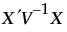, where V -1 is the inverse of the variance matrix of the responses. For further details, see Goos, 2002.
 • D-Optimality
 • Bayesian D-Optimality
 • I-Optimality
 • Bayesian I-Optimality
 • Alias Optimality
 • screening designs
 • experiments that focus on estimating effects or testing for significance
 • designs where identifying the active factors is the experimental goal
Specifically, a D-optimal design maximizes D, where D is defined as follows:
and where X is the model matrix as defined in Save X Matrix.
D-optimal split-plot designs maximize D, where D is defined as follows:
Bayesian D-optimality is a modification of the D-optimality criterion. The Bayesian D-optimality criterion is useful when there are potentially active interactions or non-linear effects. See DuMouchel and Jones (1994) and Jones et al (2008).
 • X is the model matrix as defined in Save X Matrix
 • K is a diagonal matrix with values as follows:
 ‒ k = 0 for Necessary terms
 ‒ k = 1 for If Possible main effects, powers, and interactions involving a categorical factor with more than two levels
 ‒ k = 4 for all other If Possible terms
The prior distribution imposed on the vector of If Possible parameters is multivariate normal, with mean vector 0 and diagonal covariance matrix with diagonal entries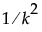. Therefore, a value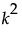is the reciprocal of the prior variance of the corresponding parameter.
The values for k are empirically determined. If Possible main effects, powers, and interactions with more than one degree of freedom have a prior variance of 1. Other If Possible terms have a prior variance of 1/16. In the notation of DuMouchel and Jones, 1994,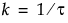.
To control the weights for If Possible terms, select Advanced Options > Prior Parameter Variance from the red triangle menu. See Advanced Options > Prior Parameter Variance.
 • predict a response
 • determine optimum operating conditions
 • determine regions in the design space where the response falls within an acceptable range
where X is the model matrix as defined in Save X Matrix.
I-optimal designs minimize the integral I of the prediction variance over the entire design space, where I is given as follows:
Here M is the moments matrix:
See Save X Matrix. For further details, see Goos and Jones (2011).
The moments matrix does not depend on the design and can be computed in advance. The row vector f (x)’ consists of a 1 followed by the effects corresponding to the assumed model. For example, for a full quadratic model in two continuous factors, f (x)’ is defined as follows:
 • X is the model matrix, defined in Save X Matrix
 • K is a diagonal matrix with values as follows:
 ‒ k = 0 for Necessary terms
 ‒ k = 1 for If Possible main effects, powers, and interactions involving a categorical factor with more than two levels
 ‒ k = 4 for all other If Possible terms
The prior distribution imposed on the vector of If Possible parameters is multivariate normal, with mean vector 0 and diagonal covariance matrix with diagonal entries. (See Bayesian D-Optimality for more details about the values k.)
where M is the moments matrix. See Save X Matrix.
Specifically, let X1 be the model matrix corresponding to the terms in the assumed model, as defined in Save X Matrix. The design defines the model that corresponds to the alias effects. Denote the matrix of model terms for the alias effects by X2.
The alias matrix is the matrix A, defined as follows:
The sum of squares of the entries in A provides a summary measure of bias. This sum of squares can be represented in terms of a trace as follows:
Let X denote the design, or model, matrix for a given assumed model with p parameters. For the definition of the model matrix, see Save X Matrix. Let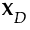denote the model matrix for a D-optimal design for the assumed model. Then the D-efficiency of the design given by X is as follows:
Custom Design constructs a design that seeks to optimize one of several optimality criteria. (See Optimality Criteria.) To optimize the criterion, Custom Design uses the coordinate-exchange algorithm (Meyer and Nachtsheim, 1995). The algorithm begins by randomly selecting values within the specified design region for each factor and each run to construct a starting design.
 • The current value of each factor is replaced by its two most extreme values.
 • The optimality criterion is computed for both of these replacements.
 • If one of the values increases the optimality criterion, this value replaces the old value.
The design obtained using this process is optimal in a large class of neighboring designs. But it is only locally optimal. To improve the likelihood of finding a globally optimal design, the coordinate-exchange algorithm is repeated a large number of times. Goos and Jones (2011, p. 36) recommend using at least 1,000 random starts for all but the most trivial design situations. The number of starting designs is controlled by the Number of Starts option. See Number of Starts. Custom Design provides the design that maximizes the optimality criterion among all the constructed designs.